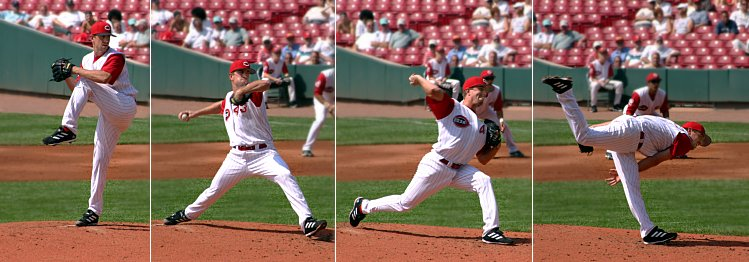# Work

Work is the transfer of mechanical energy from one object to another. Since work is a movement of energy, it is measured in the same units as energy: joules (J). The definition of work in a physics context is quite different from how it is used in a person's daily life and is as follows:

Work is done when a force is applied to an object through a distance.

This means that when a force is applied to an object through a distance, the object's total energy will be affected. The object will either speed up or slow down, resulting in a change in its kinetic energy (seen in Figure 1), or it will have an altered potential energy if, for example, it was lifted a certain height under the force of gravity.Figure 1. A pitcher does work on a baseball in order to increase its kinetic energy. His arm goes back as far as possible and then as forward as possible in order to maximize the distance the force was applied.

Work also extends beyond what a person can physically see. It can also affect the microscopic properties of a system, such as temperature. In 1843 this idea began to be explored by scientists, and its results led to the formulation of what is now known as thermodynamics. Doing work on a system can affect its internal energy, just like adding heat can. However, the two processes are fundamentally different, and can be explored on the heat vs work page.

All of the cases described so far of how work can affect a system can be summed up in a single equation:

This equation says that work () can change () a system's kinetic energy (), potential energy (), thermal energy (), or any combination of the three.

The actual work done can be calculated using the following formula:

Where

• is work, or a change in mechanical energy, measured in joules (J)
• is force measured in newtons (N)
• is displacement of the object

The arrows above force and displacement indicate that they are vectors. This means that they have an associated direction with them, which have important implications for how much work is done to an object. If both directions are the same, as they are in Figure 1, the system's energy will increase meaning positive work was done. If the directions are opposite, such as the force applied by friction and air drag to a moving car, the system's energy will decrease resulting in negative work done.

In the physics sense, work is never something an object has. It is only something that one object does to another. Work changes the amount of mechanical and internal energy possessed by objects. When work is done on a system or object, energy is added to it. When work is done by a system or object, it gives some of its energy to something else.

Throwing a ball means a hand applies a force as an arm swings forward. By applying a force on the ball over this distance, the hand is doing work on the ball, and the ball gains kinetic energy. This is what gives it speed.

The mathematical relationships between total work and total energy are described by the work-energy theorem and conservation of energy. Simple machines can change the amount of force that is necessary to move an object, but the force must be applied through a larger distance; they don't change the amount of work done.